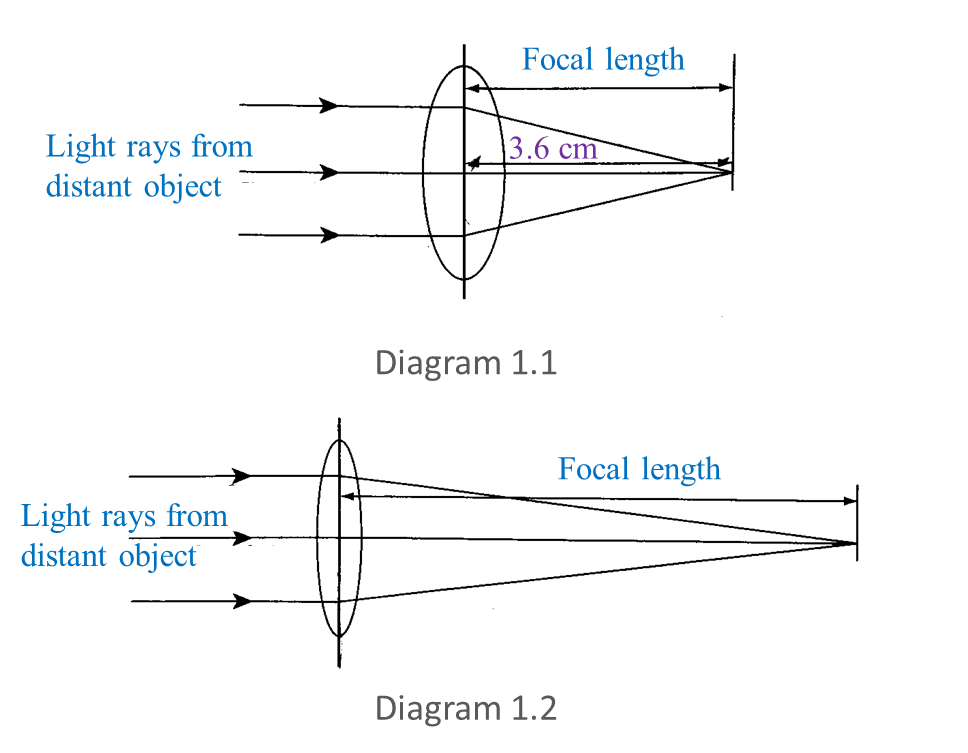# The Formation of Images by Plane Mirrors and Lenses (Structured Questions)

Question 1:
Diagram 1.1 and Diagram 1.2 show an experiment to measure the focal length of two convex lenses.(a) Measure and write down the focal length in Diagram 1.1.
(b) Observe Diagram 1.1 and 1.2.
What is the difference between the focal lengths of the two convex lenses?
(c) State one inference for this experiment.
(d) State the variables in this experiment.
(i) Constant variable
(ii) Manipulated variable